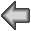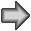Demos
Source Code of hanoi.numDocument

` The Towers of Hanoi.
` The function 'hanoi' is the recursive algorithm that
` actually solves the problem. All the other functions
` are used for displaying the solution graphically.
` Note: to change the speed of the animation modify the
` parameter 'del' below (smaller value = slower).

`------------------------------------------------------------
` The recursive function (Hanoi algorithm).
` Note how 'hanoi' calls itself to solve the same task
` with one ring less.

func hanoi(n,s,d,i) ` move n rings from s to d using iif n > 0hanoi(n-1,s,i,d) ` move n-1 rings from s to i using d  move(n,s,d)      ` move ring n from s to d  hanoi(n-1,i,d,s) ` move the n-1 rings from i to d using s

`------------------------------------------------------------
` move from post p to post q

func move(i,p,q)up(i,p)   ` take out  down(i,q) ` put down

`------------------------------------------------------------
` move ring 'i' up post 'p'

func up(i,p)h = %ph[p]+%del ` initial ring height
while h < %ymaxputring(i,p,h)  refresh ` update viewer  h += %del
putring(i,p,%ymax) ` final ring height
refresh     ` update viewer
%ph[p] -= 1 ` new post height: decrease by 1

`------------------------------------------------------------
` move ring 'i' down post 'p'

func down(i,p)h = %ymax ` initial ring height
while h > %ph[p]putring(i,p,h)  refresh ` update viewer  h -= %del
putring(i,p,%ph[p]) ` final ring height
refresh     ` update viewer
%ph[p] += 1 ` new post height: increase by 1

`------------------------------------------------------------
` define xr,yr: coordinates of ring i on post p at height h

func putring(i,p,h)w = 6-%n+i ` width  %xr[i,*] = -w,w  %xr[i,*] *= 1.5  %xr[i,*] += (p-2)*%xmax  %yr[i,*] = h+1.3

`------------------------------------------------------------
` put n rings on post p

func init(n,p)clear %ph  for j = n downto 1 down(j,p)

`------------------------------------------------------------
` initializations

n = 4 ` n rings (up to 6)
s = 1 ` source: from post 1
d = 3 ` destination: to post 3
i = 2 ` intermediate: trough post 2

del = 0.25 ` ring vertical movement pitch

xmax = 24  ` distance between center post to side posts
ymin = 0.3 ` lowest ring position
ymax = 6   ` highest ring position

x1 = -xmax ` location of post 1
x2 = 0     ` location of post 2
x3 = xmax  ` location of post 3
y = ymin,ymax ` post height range
xbase = -1.5*xmax,1.5*xmax ` base width range
ybase = ymin  ` y position of base
ph:0   ` array: current heights of posts 1 to 3
xr[n,2]:0 ` ring x-coordinates
yr[n,2]:0 ` ring y-coordinates

`------------------------------------------------------------
` call hanoi in a loop until interrupted by
` user (Pause or Stop)

loopinit(n,s)      ` put n rings on post s  wait 1         ` stand by  hanoi(n,s,d,i) ` move rings from s to d through i  beep  wait 3  ` rest

Demos